x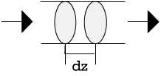Plug flowOverview

In fluid mechanics
Fluid mechanics
Fluid mechanics is the study of fluids and the forces on them. Fluid mechanics can be divided into fluid statics, the study of fluids at rest; fluid kinematics, the study of fluids in motion; and fluid dynamics, the study of the effect of forces on fluid motion...

, plug flow is a simple model of the velocity profile of a fluid
Fluid
In physics, a fluid is a substance that continually deforms under an applied shear stress. Fluids are a subset of the phases of matter and include liquids, gases, plasmas and, to some extent, plastic solids....

flowing
Fluid dynamics
In physics, fluid dynamics is a sub-discipline of fluid mechanics that deals with fluid flow—the natural science of fluids in motion. It has several subdisciplines itself, including aerodynamics and hydrodynamics...

in a pipe
Pipe (material)
A pipe is a tubular section or hollow cylinder, usually but not necessarily of circular cross-section, used mainly to convey substances which can flow — liquids and gases , slurries, powders, masses of small solids...

. In plug flow, the velocity of the fluid is assumed to be constant across any cross-section of the pipe perpendicular to the axis of the pipe. The plug flow model assumes there is no boundary layer
Boundary layer
In physics and fluid mechanics, a boundary layer is that layer of fluid in the immediate vicinity of a bounding surface where effects of viscosity of the fluid are considered in detail. In the Earth's atmosphere, the planetary boundary layer is the air layer near the ground affected by diurnal...

adjacent to the inner wall of the pipe.

The plug flow model has many practical applications. One example is in the design of chemical reactor
Chemical reactor
In chemical engineering, chemical reactors are vessels designed to contain chemical reactions. The design of a chemical reactor deals with multiple aspects of chemical engineering. Chemical engineers design reactors to maximize net present value for the given reaction...

s.Models of Plug Flow ReactorEncyclopedia
In fluid mechanics
Fluid mechanics
Fluid mechanics is the study of fluids and the forces on them. Fluid mechanics can be divided into fluid statics, the study of fluids at rest; fluid kinematics, the study of fluids in motion; and fluid dynamics, the study of the effect of forces on fluid motion...

, plug flow is a simple model of the velocity profile of a fluid
Fluid
In physics, a fluid is a substance that continually deforms under an applied shear stress. Fluids are a subset of the phases of matter and include liquids, gases, plasmas and, to some extent, plastic solids....

flowing
Fluid dynamics
In physics, fluid dynamics is a sub-discipline of fluid mechanics that deals with fluid flow—the natural science of fluids in motion. It has several subdisciplines itself, including aerodynamics and hydrodynamics...

in a pipe
Pipe (material)
A pipe is a tubular section or hollow cylinder, usually but not necessarily of circular cross-section, used mainly to convey substances which can flow — liquids and gases , slurries, powders, masses of small solids...

. In plug flow, the velocity of the fluid is assumed to be constant across any cross-section of the pipe perpendicular to the axis of the pipe. The plug flow model assumes there is no boundary layer
Boundary layer
In physics and fluid mechanics, a boundary layer is that layer of fluid in the immediate vicinity of a bounding surface where effects of viscosity of the fluid are considered in detail. In the Earth's atmosphere, the planetary boundary layer is the air layer near the ground affected by diurnal...

adjacent to the inner wall of the pipe.

The plug flow model has many practical applications. One example is in the design of chemical reactor
Chemical reactor
In chemical engineering, chemical reactors are vessels designed to contain chemical reactions. The design of a chemical reactor deals with multiple aspects of chemical engineering. Chemical engineers design reactors to maximize net present value for the given reaction...

s. Essentially no back mixing is assumed with "plugs" of fluid passing through the reactor. This results in differential equation
Differential equation
A differential equation is a mathematical equation for an unknown function of one or several variables that relates the values of the function itself and its derivatives of various orders...

s that need to be integrated to find the reactor conversion and outlet temperatures. Other simplifications used are perfect radial mixing and a homogeneous bed structure.

An advantage of the plug flow model is that no part of the solution of the problem can be perpetuated "upstream". This allows one to calculate the exact solution to the differential equation knowing only the initial conditions. No further iteration is required. Each "plug" can be solved independently provided the previous plug's state is known.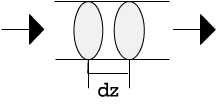The flow model in which the velocity profile consists of the fully developed boundary layer
Boundary layer
In physics and fluid mechanics, a boundary layer is that layer of fluid in the immediate vicinity of a bounding surface where effects of viscosity of the fluid are considered in detail. In the Earth's atmosphere, the planetary boundary layer is the air layer near the ground affected by diurnal...

is known as pipe flow
Pipe flow
Pipe flow, a branch of Hydraulics and Fluid Mechanics, is a type of liquid flow within a closed conduit . The other type of flow within a conduit being open channel flow....

. In laminar pipe flow
Laminar flow
Laminar flow, sometimes known as streamline flow, occurs when a fluid flows in parallel layers, with no disruption between the layers. At low velocities the fluid tends to flow without lateral mixing, and adjacent layers slide past one another like playing cards. There are no cross currents...

, the velocity profile is parabolic
Parabola
In mathematics, the parabola is a conic section, the intersection of a right circular conical surface and a plane parallel to a generating straight line of that surface...

.

## Determination of Plug Flow

For flows in pipes, if flow is turbulent then the laminar sublayer
Laminar sublayer
The laminar sublayer, also called the viscous sublayer, is the region of a mainly-turbulent flow that is near a no-slip boundary and in which the flow is laminar. The existence of the laminar sublayer can be understood in that the flow velocity decreases towards the no-slip boundary...

caused by the pipe wall will is so thin that it is negligible. Plug flow will be achieved if the sublayer thickness is much less than the pipe diameter (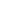<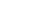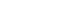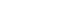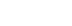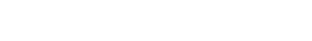where f is the Darcy friction factor (from the above equation or the Moody Chart
Moody chart
The Moody chart or Moody diagram is a graph in non-dimensional form that relates the Darcy friction factor, Reynolds number and relative roughness for fully developed flow in a circular pipe...

),is the sublayer
Sublayer
In telecommunication, the term sublayer has the following meanings:# In a layered open communications system, a specified subset of the services, functions, and protocols included in a given layer....

thickness, D is the pipe diameter,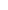is the density
Density
The mass density or density of a material is defined as its mass per unit volume. The symbol most often used for density is ρ . In some cases , density is also defined as its weight per unit volume; although, this quantity is more properly called specific weight...

, u* is the friction velocity (not an actual velocity of the fluid),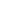is the shear on the wall, and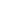is the pressure loss down the length L of the pipe.is the relative roughness of the pipe.
In this regime the pressure drop is a result of inertia-dominated turbulent shear stress rather than viscosity-dominated laminar shear stress.